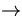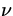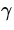It is found that nuclei with mass numbers greater than about 100 spontaneously decay into other types of nuclei. Such nuclei are said to be radioactive, and there are three main types of such decays.
• alpha decay, which occurs by emission of an alpha ( 42 He) nuclei. An example of this is the decay of Uranium:

 23892 U23490 Th + 42 He (5)

• beta decay, which occurs by emission of a beta particle (electron or positron). An example of this is the decay of Nitrogen:

 127 N126 C + 01 e +(6)

The notation 01 e denotes an electron/positron - a positron is identical except it has a positive charge - whileis a neutrino, which is a massless, electrically neutral particle.
• gamma decay, which occurs by emission of gamma particles (photons, or quanta of light). An example of this is the decay of a Carbon atom in an excited state:

 126 C *126 C +(7)

wheredenotes the photon.
As with the previous discussion of binding energy, it is found that the mass of the decaying particle on the left-hand-side of these equations is greater than the sum of the masses of the decay products on the right-hand-sides. This loss'' in mass is interpreted, through Eq. (29.2), as a conversion into other forms of energy, mainly kinetic energy of the products. This will be illustrated in some examples later. Although certainly dangerous to life, low-level radioactivity has some important applications in our everyday life, including carbon dating and smoke detectors.Next: Measuring Radioactivity Up: Nuclear Physics Previous: Nuclei